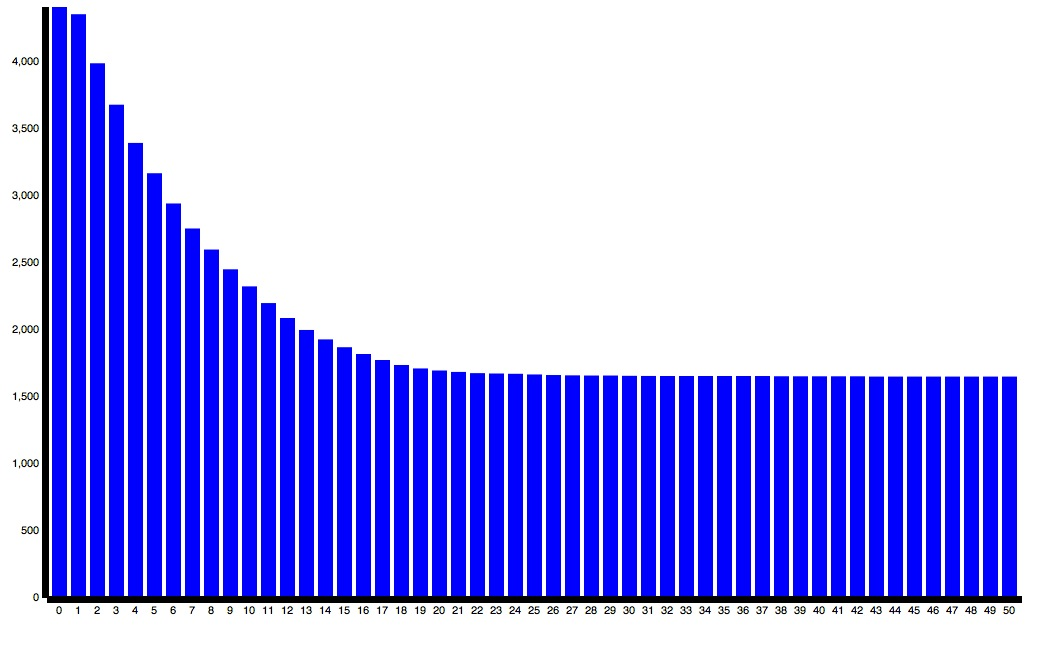Nourishing Pear Medley

# npm

Wondering what’s next for npm?Check out our public roadmap! »

## algorithmbox

1.0.1 • Public • Published

## AlgorithmBox

AlgorithmBox is an algorithm development toolkit for solving discrete optimization problems. It provides a framework for implementing metaheuristic algorithms in javascript and an empirical experiment library for testing the algorithm against a number of standard optimization problems. It can be installed as a Node.js module via

## How to Use

With algorithmbox, you can write a few lines of javascript code to implement a local search algorithm and run it against problems such as TSP and SAT. AlgorithmBox defined a number of basic stochastic local search algorithms including

It also provides spec for the following optimization problems

• Travelling Sales Problem (TSP)
• Satisfiability Problem (SAT)

To implement a hill climbing algorithm for solving TSP, do the following

To load a TSP problem instance from a file such as tsplib, do

Now run the algorithm against the problem instance

## Experiment and Analysis

AlgorithmBox provides a simple framework for experimenting with different problem instances and algorithm parameter configurations . To define an experiment

To run the experiment and output the raw data result to a folder:

To analyze the experimental result, use the Analyzer to load the experiment raw data

AlgorithmBox provides the following experimental analysis matrix

• Runtime Quality Distribution showing how solution quality changes over local search steps
• Runtime Solvability Distribution showing the probability of reaching a solution quality over local search steps
• Problem Solution Quality shows the average solution of the algorithm across mutlitple problem instances over a number of indepedent runs

For further details, please look into the test case examples.

# Visualization and Ploting

A sample visualization is provided (test/test_visualization.js) that demonstrate how to use Socket.IO and D3 to visualize the runtime quality distribution of a typical experiment. In the test folder, do

In the browser, open

and you would obtain the runtime quality distribution showing how solution quality (Y Axis for minimization problem) improves over runtime (X axis as local search steps)AlgorithmBox is still a new concept under developement. Contributors are welcomed.

## Author

Denny C. Dai (dennycd@me.com http://dennycd.me)

## Keywords

none

### Install

`npm i algorithmbox`

7

1.0.1

none